# Can

Watering can full of water weighs 10 kg. Half-full can weighs 5.5 kg. How much weigh can?

k =  1 kg

### Step-by-step explanation:

k+v = 10
k + v/2 = 5.5

k+v = 10
2k+v = 11

k = 1
v = 9

Our linear equations calculator calculates it.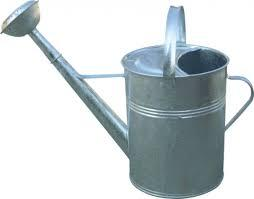Did you find an error or inaccuracy? Feel free to write us. Thank you!Tips to related online calculators
Do you have a linear equation or system of equations and looking for its solution? Or do you have a quadratic equation?

## Related math problems and questions:

• Watering can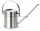A watering can full of water weighs 11 kg, the weight of water is 10 kg greater than the weight of an empty watering can, how much is the weight of the watering can?
• Bag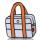Nelly found interesting point. An empty bag weighs 4 kg less than full. And empty bag is 5 times easier than full. How many kg weigh things in this bag?
• BucketThe bucket half filled with water weighs 5.55 kg, the full bucket weighs 9.85 kg. How much does the bucket weigh?
• Children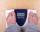Margaret and Zdena weigh the same and Petra 3 kg more. Together weigh 156 kg. How much do they weigh?
• HomeworkMother and Kate together weigh 24.5 kg more than the father. Father weighs 7.6 kg more than mother. Father weight 44.8 kg more than Kate. Determine weights of father mother and Kate.
• Barrel 4Barrel of water weighs 63 kg. After off 75% water, the weight of the barrel with water is 21 kg. How many kg weigh empty barrel and how many kgs water in it?
• Brick weightThe brick weighs 2 kg and a half bricks. How much does one brick weigh?
• The water barrel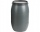The water barrel weighs 122 kg. If we pour 75% of the water out of it, it will weigh 35 kg. What is the weight of the barrel?
• Water wessel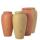The vessel containing water has a weight 19 kg. After pouring half the water its weigth 11 kg. What is the weight of the empty vessel?
• Barrel 2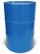Empty barrel weighs 7 kg. When it filled with water up to 45% height, weighs 60 kg. How heavy is barrel full of water?
• Water containerContainer with water weighs 1.48 kg. When we cast 75% of water container of water weight 0.73 kg. How heavy is an empty container?
• One kilogramThe apple weighs 125 grams and half apple. How many apples weigh 1 kilogram?
• The barrel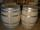The barrel is 135 cm high. If we fill it to a height of 60 cm and it weighs 163 kg. If we fill it to a height of 80 cm, it weighs 213 kg. How many kg weighs a full and how much empty barrel?
• Reservoir + waterThe reservoir filled with water weighs 12 kg and after pouring off three-quarters of the water weights 3 kg. Calculate the weight and volume of the reservoir.
• Two bricks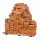One brick weighs 1kg and half a brick, how many weigh two bricks?
• Bricks II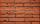3/4 bricks weighs 6 kg and 2/3 bricks. How weighs one whole brick?
• Water in body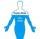Water in the human organism of all the compounds represented constitutes about 3/5 of the total weight. How many kilograms of water is in your body when you weigh 51 kg?